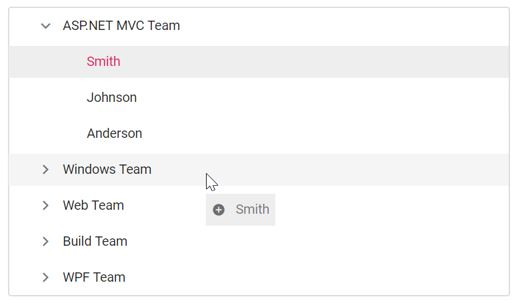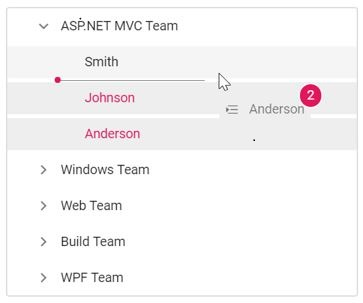# Drag and Drop

25 Feb 202224 minutes to read

The TreeView control allows you to drag and drop any node by setting allowDragAndDrop to true. Nodes can be dragged and dropped at all levels of the same TreeView.

The dragged nodes can be dropped at any level by indicator lines with line, plus/minus, and restrict icons. It represents the exact position where the node is to be dropped as sibling or child.

The following table explains the usage of indicator icons.

Icons Description
Plus icon Indicates that the dragged node is to be added as child of target node.
Minus or restrict icon Indicates that the dragged node is not to be dropped at the hovered region.
In between icon Indicates that the dragged node is to be added as siblings of hovered region.
• If you need to prevent dragging action for a particular node, the `nodeDragStart` event can be used which is triggered when the node drag is started. If you need to prevent dropping action for a particular node, the `nodeDragStop` event can be used which is triggered when the drag is stopped.

• The `nodeDragging` event is triggered when the TreeView node is being dragged. You can customize the cloned element in this event.

• The `nodeDropped` event is triggered when the TreeView node is dropped on the target element successfully.

In the following sample, the allowDragAndDrop property is enabled.

``````<ejs-treeview  id="treedata" allowDragAndDrop="true">
<e-treeview-fields dataSource="ViewBag.dataSource" id="id" parentId="pid" text="name" hasChildren="hasChild" expanded="expanded" selected="is_selected"></e-treeview-fields>
</ejs-treeview>``````
``````public IActionResult DragDrop()
{
List<object> treedata = new List<object>();
{
id = 1,
name = "ASP.NET MVC Team",
hasChild = true,
expanded = true
});
{
id = 2,
pid = 1,
name = "Smith",
is_selected=true

});
{
id = 3,
pid = 1,
name = "Johnson",
is_selected = true
});

{
id = 4,
pid = 1,
name = "Anderson"
});
{
id = 6,
hasChild = true,
name = "Windows Team",

});
{
id = 7,
pid=6,
name="Clark"

});
{
id = 8,
pid = 6,
name = "Wright"
});
{
id = 9,
pid = 6,
name = "Lopez"
});
{
id = 10,
hasChild = true,
name = "Web Team"
});
{
id = 11,
pid=10,
name = "Joshua",

});
{
id = 12,
pid = 10,
name = "Matthew"
});
{
id = 13,
pid = 10,
name = "David"
});
{
id = 14,
hasChild = true,
name = "Build Team"
});
{
id = 15,
pid = 14,
name = "Ryan"

});
{
id = 16,
pid=14,
name = "Justin",

});
{
id = 17,
pid = 14,
name = "Robert"

});
{
id = 18,
hasChild=true,
name = "WPF Team"

});
{
id = 19,
pid = 18,
name = "Brown"

});
{
id = 20,
pid = 18,
name = "Johnson"
});
{
id = 21,
pid = 18,
name = "Miller"
});

ViewBag.dataSource = treedata;
return View();
}``````

Output be like the below.## Multiple-node drag and drop

To drag and drop more than one node, you should enable the allowMultiSelection property along with the `allowDragAndDrop` property.

To perform multi-selection, press and hold CTRL key and click the desired nodes. To select range of nodes, press and hold the SHIFT key and click the nodes.

In the following sample, the `allowMultiSelection` property is enabled along with the `allowDragAndDrop` property.

``````<ejs-treeview id="treedata" allowDragAndDrop="true" allowMultiSelection="true">
<e-treeview-fields dataSource="ViewBag.dataSource" id="id" parentId="pid" text="name" hasChildren="hasChild" expanded="expanded" selected="is_selected"></e-treeview-fields>
</ejs-treeview>``````
``````public IActionResult DragDrop()
{
List<object> treedata = new List<object>();
{
id = 1,
name = "ASP.NET MVC Team",
hasChild = true,
expanded = true
});
{
id = 2,
pid = 1,
name = "Smith",
is_selected=true

});
{
id = 3,
pid = 1,
name = "Johnson",
is_selected = true
});

{
id = 4,
pid = 1,
name = "Anderson"
});
{
id = 6,
hasChild = true,
name = "Windows Team",

});
{
id = 7,
pid=6,
name="Clark"

});
{
id = 8,
pid = 6,
name = "Wright"
});
{
id = 9,
pid = 6,
name = "Lopez"
});
{
id = 10,
hasChild = true,
name = "Web Team"
});
{
id = 11,
pid=10,
name = "Joshua",

});
{
id = 12,
pid = 10,
name = "Matthew"
});
{
id = 13,
pid = 10,
name = "David"
});
{
id = 14,
hasChild = true,
name = "Build Team"
});
{
id = 15,
pid = 14,
name = "Ryan"

});
{
id = 16,
pid=14,
name = "Justin",

});
{
id = 17,
pid = 14,
name = "Robert"

});
{
id = 18,
hasChild=true,
name = "WPF Team"

});
{
id = 19,
pid = 18,
name = "Brown"

});
{
id = 20,
pid = 18,
name = "Johnson"
});
{
id = 21,
pid = 18,
name = "Miller"
});

ViewBag.dataSource = treedata;
return View();
}``````

Output be like the below.